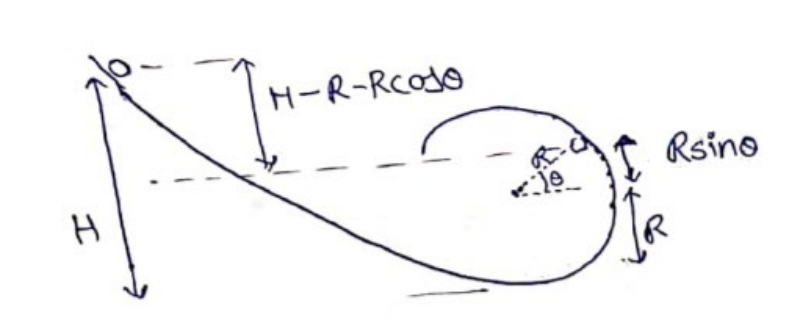Deepak Scored 45->99%ile with Bounce Back Crack Course. You can do it too!

# Solve the following :

Question:

Figure shows a small spherical ball of mass $m$ rolling down the loop track. The ball is released on the linear portion at a vertical height $\mathrm{H}$ from the lowest point. The circular part shown has a radius $\mathrm{R}$. (a) Find the kinetic energy of the ball when it is at a point $\mathrm{A}$ where the radius makes an angle $\theta$ with the horizontal.

(b) Find the radial and the tangential accelerations of the centre when the ball is at $A$.

(c) Find the normal force and the frictional force acting on the ball if $\mathrm{H}=60 \mathrm{~cm}, \mathrm{R}=10 \mathrm{~cm}, \theta=0$ and $\mathrm{m}=70 \mathrm{~g}$.

Solution:Energy conservation

$K \cdot E_{i}+P \cdot E_{i}=K \cdot E_{f}+P_{x} E_{f}$

$0+m g(H-R-R \sin \theta)=K . E_{A}$

(b)

$K_{\cdot} E_{A}=\frac{1}{2} I \omega^{2}+\frac{1}{2} m v^{2}$

$=\frac{1}{2}\left(\frac{2}{5} m v^{2}\right)\left(\frac{v^{2}}{v^{2}}\right)+\frac{1}{2} m v^{2}$

$K . E_{A}=\frac{7}{10} m v^{2}=m g(H-R-R \sin \theta)$

$v^{2}=\frac{10}{7} g(H-R-R \sin \theta)$

$a_{r}=\frac{v^{2}}{R}=\frac{10 g}{7 R}(H-R-R \sin \theta)$

Differentiate equation (i) for tangential acceleration

$2 v \frac{d v}{d t}=\frac{-10 g}{7} R \cos \theta \cdot \frac{d \theta}{d t}$

$\frac{d v}{d t}=\frac{-5}{7} g \cos \theta \cdot \frac{R \omega}{v}$

$a_{t}=\frac{-5}{7} g \cos \theta$

Horizontal direction

$N=m a_{r}=m \times \frac{10 g}{7} \frac{(H-R-R \sin \theta)}{R}$ $=\left(\frac{70}{1000}\right)\left(\frac{10}{7} \times 10\right)\left(\frac{0.6-0.1-0.1 \times \sin 0^{\circ}}{0.1}\right)$ $\mathrm{N}=5$ Vertical direction $m g-f f=m a_{t}$ $f f=m\left(g-a_{t}\right)$

$m g-f f=m a_{t}$

$=\frac{70}{1000}\left(10-\frac{5}{7} \times 10\right)$

$f f=0.2 \mathrm{~N}$